International
Tables for
Crystallography
Volume B
Reciprocal space
Edited by U. Shmueli

International Tables for Crystallography (2006). Vol. B, ch. 5.1, p. 536   | 1 | 2 |

## Section 5.1.2.4. Fundamental equations of dynamical theory

A. Authiera*

aLaboratoire de Minéralogie-Cristallographie, Université P. et M. Curie, 4 Place Jussieu, F-75252 Paris CEDEX 05, France
Correspondence e-mail: authier@lmcp.jussieu.fr

#### 5.1.2.4. Fundamental equations of dynamical theory

| top | pdf |

In order to obtain the solution of dynamical theory, one inserts expansions (5.1.2.15)and (5.1.2.4)into the propagation equation (5.1.2.2). This leads to an equation with an infinite sum of terms. It is shown to be equivalent to an infinite system of linear equations which are the fundamental equations of dynamical theory. Only those terms in (5.1.2.15)whose wavevector magnitudesare very close to the vacuum value, k, have a non-negligible amplitude. These wavevectors are associated with reciprocal-lattice points that lie very close to the Ewald sphere. Far from any Bragg reflection, their number is equal to 1 and a single plane wave propagates through the medium. In general, for X-rays, there are only two reciprocal-lattice points on the Ewald sphere. This is the so-called two-beam case to which this treatment is limited. There are, however, many instances where several reciprocal-lattice points lie simultaneously on the Ewald sphere. This corresponds to the many-beam case which has interesting applications for the determination of phases of reflections [see, for instance, Chang (1987)and Hümmer & Weckert (1995)]. On the other hand, for electrons, there are in general many reciprocal-lattice points close to the Ewald sphere and many wavefields are excited simultaneously (see Chapter 5.2).

In the two-beam case, for reflections that are not highly asymmetric and for Bragg angles that are not close to, the fundamental equations of dynamical theory reduce to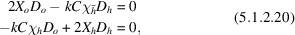where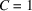if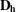is normal to the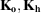plane and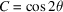iflies in the plane; this is due to the fact that the amplitude with which electromagnetic radiation is scattered is proportional to the sine of the angle between the direction of the electric vector of the incident radiation and the direction of scattering (see, for instance, IT C, Section 6.2.2). The polarization of an electromagnetic wave is classically related to the orientation of the electric vector; in dynamical theory it is that of the electric displacement which is considered (see Section A5.1.1.3of the Appendix).

The system (5.1.2.20)is therefore a system of four equations which admits four solutions, two for each direction of polarization. In the non-absorbing case, to a very good approximation,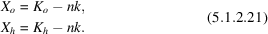In the case of an absorbing crystal,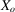andare complex. Equation (5.1.5.2)gives the full expression for.

### References

Chang, S.-L. (1987). Solution to the X-ray phase problem using multiple diffraction – a review. Crystallogr. Rev. 1, 87–189.Google Scholar
Hümmer, K. & Weckert, E. (1995). Enantiomorphism and three-beam X-ray diffraction: determination of the absolute structure. Acta Cryst. A51, 431–438.Google Scholar